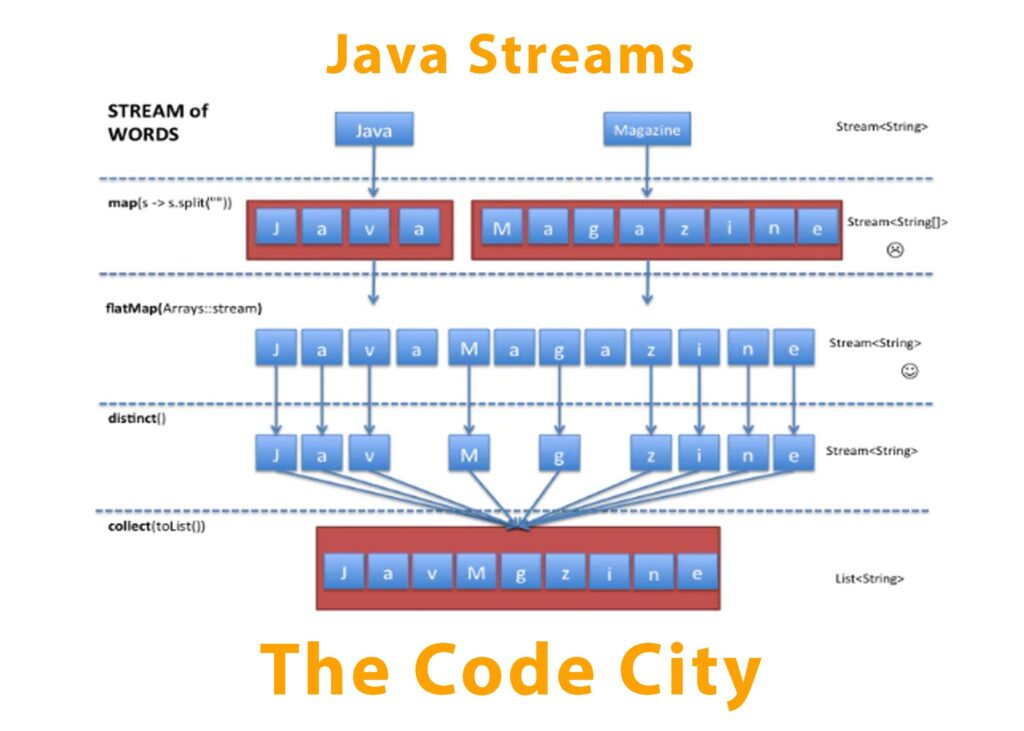# Streams in Java – Quick Guide with Examples

Java introduced Stream API in java 8, which is useful to perform operations on collections. Java streams have definitely made processing collections and life easier. But in order to work with streams, it’s important to understand following:

1. Functional programming
2. Functional Interface
3. Lambda Expression

Here I am going to explain all three concepts to help you understand Java Streams briefly.

## Underlying Concepts

1. Functional Programming: Let’s start with functional programming, it’s a way of programming which is declarative, which means we write what we want instead to how to achieve that. Let’s take a simple example where we want to filter a list to get a list of strings starting with ‘J’. This is how our code would look like before streams. This is called imperative programming as we write the logic for what we want.
``````List<String> list = new ArrayList<String>(Arrays.asList("Java","Java Script","C","Python"));

List<String> filteredList = new ArrayList<String>();
for(String s:list) {
if(s.startsWith("J")) {
}
}``````

This was lot compared to functional way, where we just make use of existing functions to achieve the same result. As can be seen below, it’s more declarative as we only state what we want which in our case is filter and collect, and our code is pretty concise.

```List<String> filteredList1 = list.stream().filter(s->s.startWith("J"))
.collect(Collectors.toList());```

2. Functional Interfaces: A functional interface is an interface which have exactly one abstract method. Runnable is a functional interface as it contains only one abstract method run(). Let’s declare a functional interface as below which have single abstract method sayHello(). You might want to take a look at the functional interfaces introduced in java 8 like Predicate, Function, Consumer, Supplier.

``` public MyInterface{
void sayHello();
}```

3. Lambda Expression: A lambda expression is an unnamed method. It is used to implement a functional interface as it contains only one method. Here’s how to define lambda expression:

``(parameter list) -> lambda body``

-> is known as lambda operator or arrow operator. we can implement MyInterface using lambda expression as below:

```()->System.out.println("Hello World!");
or
MyInterface mi = ()->System.out.println("Hello World!");```

Lambda expression can be assigned to a variable or passed as a method argument.

## Getting Into Java Streams

Now that we have a basic idea of what functional programming, functional interface and lambda expressions are, let’s take a look in detail how these are used with streams. In our example of stream above we have used lambda expression as the argument for filter().

If we take a look at the signature of filter method, it takes Predicate as the method argument, which is a functional interface with an abstract method; boolean test(T t). The implementation of this interface is the lambda expression s->s.startWith(“J”), which we have passed to filter().

All the methods available in streams api makes operations on Collection simpler. The operations performed by these methods can be divided into intermediate and terminal operations. A new stream is produced after performing intermediate operation hence, multiple intermediate operations can be performed in pipeline, conversely, stream is said to be consumed after terminal operation and further operations cannot be performed.

Examples of intermediate operations:

1. Filter: filter() is an intermediate operation and a new stream is produced after this operation.
2. Map: map() is another very common intermediate operation, which takes Function as parameter.
`List<String> listUpper = list.stream().map(s->s.toUpperCase()).collect(Collectors.toList());`

3. Sorted: sorted() is used for sorting and takes Comparator as parameter.

```List<String> sortedList = list.stream().sorted((s1,s2)->
s1.compareTo(s2)).collect(Collectors.toList());```

Example of multiple intermediate operations map() and limit() in pipeline.

```List<String> limitedList = list.stream().map(s->s.toUpperCase())
.limit(3).collect(Collectors.toList());```

There are multiple other intermediate operations like disctinct(), flatMap() for which descriptions can be found in Stream API official documentation.

Examples of terminal operations:

1. Collect: collect() used in all of the examples above is a terminal operation. You could also use collect to concatenate string as follows:
`String langs = list.stream().filter(s->s.startsWith("J")).collect(Collectors.joining(","));`

2. Count: count() is used to count total number of elements in stream.

`long count = list.stream().filter(s->s.startsWith("J")).count();`

3. AnyMatch: anyMatch() takes a predicate as parameter and return boolean.

`boolean match = list.stream().anyMatch(x->x.equals("Java"));`

4. Reduce: reduce() takes BinaryOperator to reduce the stream. Stream API contains set of predefined reduction operations such as average (), sum (), min (), max (), and count ().

`Optional<String> reduced = list.stream().reduce((a,b)->a+","+b);  orString reduced  = list.stream().reduce("",(a,b)->a+","+b); //reduce with initial value returns String not Optional`

Lazy Evaluation: One of the most important characteristics of Java streams is that they allow for significant optimizations through lazy evaluations. All intermediate operations are lazy, so they’re not executed until a result of a processing is required. You would note in the following example that peek only prints first element starting with ‘J’ which is ‘Java’ and wouldn’t filter other elements.

```Optional<String> first = list.stream().filter(s->s.startsWith("J"))
.peek(s1->System.out.println("Peeking :"+s1)).findFirst();```

Note: It would be excellent to go through the official documentation of Stream API to see the exhaustive list of available methods.